Main MRPT website > C++ reference for MRPT 1.5.7
mrpt::poses::CPose3DRotVec Class Reference

## Detailed Description

A 3D pose, with a 3D translation and a rotation in 3D parameterized in rotation-vector form (equivalent to axis-angle).

The 6D transformation in SE(3) stored in this class is kept in two separate containers: a 3-array for the rotation vector, and a 3-array for the translation.

pose.m_rotvec[{0:2}]=... // Rotation vector
pose.m_coords[{0:2}]=... // Translation

For a complete descriptionan of Points/Poses, see mrpt::poses::CPoseOrPoint, or refer to the 2D/3D Geometry tutorial online.

There are Lie algebra methods: exp and ln (see the methods for documentation).

CPose3DRotVec, CPoseOrPoint,CPoint3D, mrpt::math::CQuaternion

Definition at line 41 of file CPose3DRotVec.h.

#include <mrpt/poses/CPose3DRotVec.h>

Inheritance diagram for mrpt::poses::CPose3DRotVec: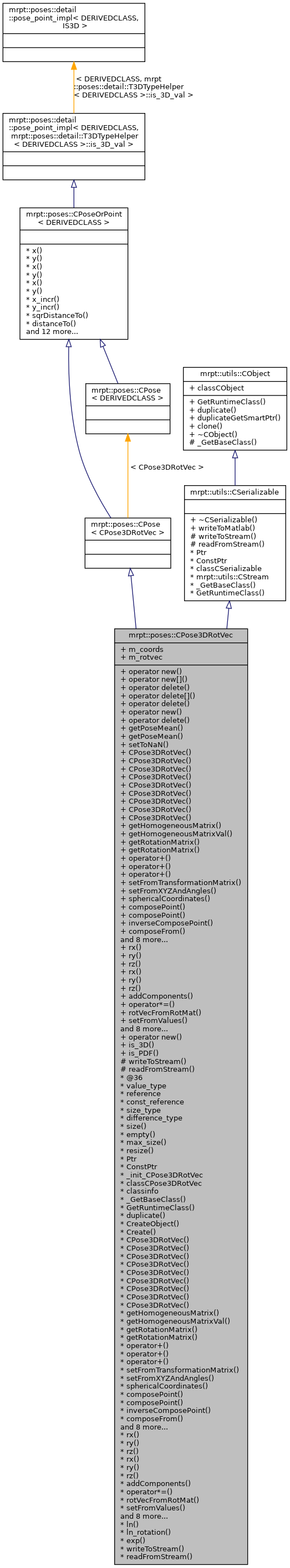## Public Types

enum  { is_3D_val = 1 }

enum  { rotation_dimensions = 3 }

enum  { is_PDF_val = 0 }

typedef CPose3DRotVec type_value
Used to emulate CPosePDF types, for example, in mrpt::graphs::CNetworkOfPoses. More...

## Public Member Functions

voidoperator new (size_t size)

voidoperator new[] (size_t size)

void operator delete (void *ptr) throw ()

void operator delete[] (void *ptr) throw ()

void operator delete (void *memory, void *ptr) throw ()

voidoperator new (size_t size, const std::nothrow_t &) throw ()

void operator delete (void *ptr, const std::nothrow_t &) throw ()

const type_valuegetPoseMean () const

type_valuegetPoseMean ()

void setToNaN () MRPT_OVERRIDE
Set all data fields to quiet NaN. More...

virtual mxArraywriteToMatlab () const
Introduces a pure virtual method responsible for writing to a mxArray Matlab object, typically a MATLAB struct whose contents are documented in each derived class. More...

mrpt::utils::CObjectPtr duplicateGetSmartPtr () const
Returns a copy of the object, indepently of its class, as a smart pointer (the newly created object will exist as long as any copy of this smart pointer). More...

CObjectclone () const
Cloning interface for smart pointers. More...

Constructors
CPose3DRotVec ()
Default constructor, with all the coordinates set to zero. More...

CPose3DRotVec (TConstructorFlags_Poses constructor_dummy_param)
Fast constructor that leaves all the data uninitialized - call with UNINITIALIZED_POSE as argument. More...

CPose3DRotVec (const double vx, const double vy, const double vz, const double x, const double y, const double z)
Constructor with initilization of the pose. More...

CPose3DRotVec (const mrpt::math::CArrayDouble< 6 > &v)
Constructor with initilization of the pose from a vector [w1 w2 w3 x y z]. More...

CPose3DRotVec (const CPose3DRotVec &o)
Copy constructor. More...

CPose3DRotVec (const math::CMatrixDouble44 &m)
Constructor from a 4x4 homogeneous matrix: More...

CPose3DRotVec (const CPose3D &m)
Constructor from a CPose3D object. More...

CPose3DRotVec (const mrpt::math::CQuaternionDouble &q, const double x, const double y, const double z)
Constructor from a quaternion (which only represents the 3D rotation part) and a 3D displacement. More...

CPose3DRotVec (const double *vec6)
Constructor from an array with these 6 elements: [w1 w2 w3 x y z] where r{ij} are the entries of the 3x3 rotation matrix and t{x,y,z} are the 3D translation of the pose. More...

Access 3x3 rotation and 4x4 homogeneous matrices
void getHomogeneousMatrix (mrpt::math::CMatrixDouble44 &out_HM) const
Returns the corresponding 4x4 homogeneous transformation matrix for the point(translation) or pose (translation+orientation). More...

mrpt::math::CMatrixDouble44 getHomogeneousMatrixVal () const

void getRotationMatrix (mrpt::math::CMatrixDouble33 &ROT) const
Get the 3x3 rotation matrix. More...

const mrpt::math::CMatrixDouble33 getRotationMatrix () const

Pose-pose and pose-point compositions and operators
CPose3DRotVec operator+ (const CPose3DRotVec &b) const
The operator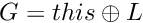is the pose compounding operator. More...

CPoint3D operator+ (const CPoint3D &b) const
The operatoris the pose compounding operator. More...

CPoint3D operator+ (const CPoint2D &b) const
The operatoris the pose compounding operator. More...

void setFromTransformationMatrix (const mrpt::math::CMatrixDouble44 &m)

void setFromXYZAndAngles (const double x, const double y, const double z, const double yaw=0, const double pitch=0, const double roll=0)

void sphericalCoordinates (const mrpt::math::TPoint3D &point, double &out_range, double &out_yaw, double &out_pitch) const
Computes the spherical coordinates of a 3D point as seen from the 6D pose specified by this object. More...

void composePoint (double lx, double ly, double lz, double &gx, double &gy, double &gz, mrpt::math::CMatrixFixedNumeric< double, 3, 3 > *out_jacobian_df_dpoint=NULL, mrpt::math::CMatrixFixedNumeric< double, 3, 6 > *out_jacobian_df_dpose=NULL) const
An alternative, slightly more efficient way of doing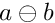with G and L being 3D points and P this 6D pose. More...

void composePoint (const mrpt::math::TPoint3D local_point, mrpt::math::TPoint3D &global_point) const
An alternative, slightly more efficient way of doingwith G and L being 3D points and P this 6D pose. More...

void inverseComposePoint (const double gx, const double gy, const double gz, double &lx, double &ly, double &lz, mrpt::math::CMatrixFixedNumeric< double, 3, 3 > *out_jacobian_df_dpoint=NULL, mrpt::math::CMatrixFixedNumeric< double, 3, 6 > *out_jacobian_df_dpose=NULL) const
Computes the 3D point L such as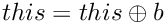. More...

void composeFrom (const CPose3DRotVec &A, const CPose3DRotVec &B, mrpt::math::CMatrixFixedNumeric< double, 6, 6 > *out_jacobian_drvtC_drvtA=NULL, mrpt::math::CMatrixFixedNumeric< double, 6, 6 > *out_jacobian_drvtC_drvtB=NULL)
Makes "this = A (+) B"; this method is slightly more efficient than "this= A + B;" since it avoids the temporary object. More...

void toQuatXYZ (CPose3DQuat &q) const
Convert this RVT into a quaternion + XYZ. More...

CPose3DRotVecoperator+= (const CPose3DRotVec &b)
Make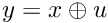(b can be "this" without problems) More...

CPose3DRotVecoperator= (const CPose3DRotVec &o)
Copy operator. More...

void inverseComposeFrom (const CPose3DRotVec &A, const CPose3DRotVec &B)
Makes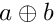this method is slightly more efficient than "this= A - B;" since it avoids the temporary object. More...

CPose3DRotVec operator- (const CPose3DRotVec &b) const
Compute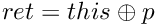. More...

void inverse ()
Convert this pose into its inverse, saving the result in itself. More...

CPose3DRotVec getInverse () const
Compute the inverse of this pose and return the result. More...

void changeCoordinatesReference (const CPose3DRotVec &p)
makes: this = p (+) this More...

Access and modify contents
double rx () const

double ry () const

double rz () const

double & rx ()

double & ry ()

double & rz ()

Scalar sum of all 6 components: This is diferent from poses composition, which is implemented as "+" operators. More...

void operator*= (const double s)
Scalar multiplication of x,y,z,vx,vy,vz. More...

mrpt::math::CArrayDouble< 3 > rotVecFromRotMat (const math::CMatrixDouble44 &m)
Create a vector with 3 components according to the input transformation matrix (only the rotation will be taken into account) More...

void setFromValues (const double x0, const double y0, const double z0, const double vx, const double vy, const double vz)
Set the pose from a 3D position (meters) and yaw/pitch/roll angles (radians) - This method recomputes the internal rotation matrix. More...

template<class ARRAYORVECTOR >
void setFrom6Vector (const ARRAYORVECTOR &vec6)
Set pose from an array with these 6 elements: [x y z vx vy vz] where v{xyz} is the rotation vector and {xyz} the 3D translation of the pose. More...

template<class ARRAYORVECTOR >
void getAs6Vector (ARRAYORVECTOR &vec6) const
Gets pose as an array with these 6 elements: [x y z vx vy vz] where v{xyz} is the rotation vector and {xyz} the 3D translation of the pose The target vector MUST ALREADY have space for 6 elements (i.e. More...

template<class ARRAYORVECTOR >
void getAsVector (ARRAYORVECTOR &v) const
Like getAs6Vector() but for dynamic size vectors (required by base class CPoseOrPoint) More...

const double & operator[] (unsigned int i) const

double & operator[] (unsigned int i)

void asString (std::string &s) const
Returns a human-readable textual representation of the object: "[x y z rx ry rz]". More...

std::string asString () const

void fromString (const std::string &s)
Set the current object value from a string generated by 'asString' (eg: "[x y z yaw pitch roll]", angles in deg. More...

## Static Public Member Functions

static voidoperator new (size_t size, void *ptr)

static bool is_3D ()

static bool is_PDF ()

## Public Attributes

mrpt::math::CArrayDouble< 3 > m_coords
The translation vector [x,y,z]. More...

mrpt::math::CArrayDouble< 3 > m_rotvec
The rotation vector [vx,vy,vz]. More...

## Static Public Attributes

static const mrpt::utils::TRuntimeClassId classCObject

RTTI stuff
static const mrpt::utils::TRuntimeClassId classCSerializable

## Protected Member Functions

CSerializable virtual methods
void writeToStream (mrpt::utils::CStream &out, int *getVersion) const
Introduces a pure virtual method responsible for writing to a CStream. More...

void readFromStream (mrpt::utils::CStream &in, int version)
Introduces a pure virtual method responsible for loading from a CStream This can not be used directly be users, instead use "stream >> object;" for reading it from a stream or "stream >> object_ptr;" if the class is unknown apriori. More...

## RTTI stuff

typedef CPose3DRotVecPtr Ptr

typedef CPose3DRotVecPtr ConstPtr

static mrpt::utils::CLASSINIT _init_CPose3DRotVec

static mrpt::utils::TRuntimeClassId classCPose3DRotVec

static const mrpt::utils::TRuntimeClassIdclassinfo

static const mrpt::utils::TRuntimeClassId_GetBaseClass ()

virtual const mrpt::utils::TRuntimeClassIdGetRuntimeClass () const
Returns information about the class of an object in runtime. More...

virtual mrpt::utils::CObjectduplicate () const
Returns a copy of the object, indepently of its class. More...

static mrpt::utils::CObjectCreateObject ()

static CPose3DRotVecPtr Create ()

## Lie Algebra methods

void ln (mrpt::math::CArrayDouble< 6 > &out_ln) const
Take the logarithm of the 3x4 matrix defined by this pose, generating the corresponding vector in the SE(3) Lie Algebra. More...

mrpt::math::CArrayDouble< 3 > ln_rotation () const
Take the logarithm of the 3x3 rotation matrix part of this pose, generating the corresponding vector in the Lie Algebra. More...

static CPose3DRotVec exp (const mrpt::math::CArrayDouble< 6 > &vect)
Exponentiate a Vector in the SE(3) Lie Algebra to generate a new CPose3DRotVec (static method). More...

## STL-like methods and typedefs

enum  { static_size = 6 }

typedef double value_type
The type of the elements. More...

typedef double & reference

typedef const double & const_reference

typedef std::size_t size_type

typedef std::ptrdiff_t difference_type

static size_type size ()

static bool empty ()

static size_type max_size ()

static void resize (const size_t n)

double x () const
Common members of all points & poses classes. More...

double & x ()

void x (const double v)

double y () const

double & y ()

void y (const double v)

void x_incr (const double v)

void y_incr (const double v)

double sqrDistanceTo (const CPoseOrPoint< OTHERCLASS > &b) const
Returns the squared euclidean distance to another pose/point: More...

double distanceTo (const CPoseOrPoint< OTHERCLASS > &b) const
Returns the Euclidean distance to another pose/point: More...

double distanceTo (const mrpt::math::TPoint3D &b) const
Returns the euclidean distance to a 3D point: More...

double distance2DToSquare (double ax, double ay) const
Returns the squared 2D distance from this pose/point to a 2D point (ignores Z, if it exists). More...

double distance3DToSquare (double ax, double ay, double az) const
Returns the squared 3D distance from this pose/point to a 3D point. More...

double distance2DTo (double ax, double ay) const
Returns the 2D distance from this pose/point to a 2D point (ignores Z, if it exists). More...

double distance3DTo (double ax, double ay, double az) const
Returns the 3D distance from this pose/point to a 3D point. More...

double norm () const
Returns the euclidean norm of vector: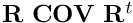. More...

mrpt::math::CVectorDouble getAsVectorVal () const
Return the pose or point as a 1xN vector with all the components (see derived classes for each implementation) More...

void getInverseHomogeneousMatrix (mrpt::math::CMatrixDouble44 &out_HM) const
Returns the corresponding 4x4 inverse homogeneous transformation matrix for this point or pose. More...

mrpt::math::CMatrixDouble44 getInverseHomogeneousMatrix () const

static bool is3DPoseOrPoint ()
Return true for poses or points with a Z component, false otherwise. More...

## ◆ const_reference

 typedef const double& mrpt::poses::CPose3DRotVec::const_reference

Definition at line 394 of file CPose3DRotVec.h.

## ◆ ConstPtr

 typedef CPose3DRotVecPtr mrpt::poses::CPose3DRotVec::ConstPtr

Definition at line 44 of file CPose3DRotVec.h.

## ◆ difference_type

Definition at line 396 of file CPose3DRotVec.h.

## ◆ Ptr

 typedef CPose3DRotVecPtr mrpt::poses::CPose3DRotVec::Ptr

A typedef for the associated smart pointer

Definition at line 44 of file CPose3DRotVec.h.

## ◆ reference

 typedef double& mrpt::poses::CPose3DRotVec::reference

Definition at line 393 of file CPose3DRotVec.h.

## ◆ size_type

 typedef std::size_t mrpt::poses::CPose3DRotVec::size_type

Definition at line 395 of file CPose3DRotVec.h.

## ◆ type_value

Used to emulate CPosePDF types, for example, in mrpt::graphs::CNetworkOfPoses.

Definition at line 378 of file CPose3DRotVec.h.

## ◆ value_type

 typedef double mrpt::poses::CPose3DRotVec::value_type

The type of the elements.

Definition at line 392 of file CPose3DRotVec.h.

## ◆ anonymous enum

 anonymous enum
Enumerator
is_3D_val

Definition at line 379 of file CPose3DRotVec.h.

## ◆ anonymous enum

 anonymous enum
Enumerator
rotation_dimensions

Definition at line 381 of file CPose3DRotVec.h.

## ◆ anonymous enum

 anonymous enum
Enumerator
is_PDF_val

Definition at line 382 of file CPose3DRotVec.h.

## ◆ anonymous enum

 anonymous enum
Enumerator
static_size

Definition at line 400 of file CPose3DRotVec.h.

## ◆ CPose3DRotVec() [1/9]

 mrpt::poses::CPose3DRotVec::CPose3DRotVec ( )
inline

Default constructor, with all the coordinates set to zero.

Definition at line 54 of file CPose3DRotVec.h.

## ◆ CPose3DRotVec() [2/9]

 mrpt::poses::CPose3DRotVec::CPose3DRotVec ( TConstructorFlags_Poses constructor_dummy_param )
inline

Fast constructor that leaves all the data uninitialized - call with UNINITIALIZED_POSE as argument.

Definition at line 60 of file CPose3DRotVec.h.

References MRPT_UNUSED_PARAM.

## ◆ CPose3DRotVec() [3/9]

 mrpt::poses::CPose3DRotVec::CPose3DRotVec ( const double vx, const double vy, const double vz, const double x, const double y, const double z )
inline

Constructor with initilization of the pose.

Definition at line 65 of file CPose3DRotVec.h.

## ◆ CPose3DRotVec() [4/9]

 mrpt::poses::CPose3DRotVec::CPose3DRotVec ( const mrpt::math::CArrayDouble< 6 > & v )
inline

Constructor with initilization of the pose from a vector [w1 w2 w3 x y z].

Definition at line 71 of file CPose3DRotVec.h.

## ◆ CPose3DRotVec() [5/9]

 mrpt::poses::CPose3DRotVec::CPose3DRotVec ( const CPose3DRotVec & o )
inline

Copy constructor.

Definition at line 77 of file CPose3DRotVec.h.

References m_coords, and m_rotvec.

## ◆ CPose3DRotVec() [6/9]

 CPose3DRotVec::CPose3DRotVec ( const math::CMatrixDouble44 & m )
explicit

Constructor from a 4x4 homogeneous matrix:

Definition at line 35 of file CPose3DRotVec.cpp.

## ◆ CPose3DRotVec() [7/9]

 CPose3DRotVec::CPose3DRotVec ( const CPose3D & m )
explicit

Constructor from a CPose3D object.

Definition at line 44 of file CPose3DRotVec.cpp.

## ◆ CPose3DRotVec() [8/9]

 CPose3DRotVec::CPose3DRotVec ( const mrpt::math::CQuaternionDouble & q, const double _x, const double _y, const double _z )

Constructor from a quaternion (which only represents the 3D rotation part) and a 3D displacement.

Definition at line 55 of file CPose3DRotVec.cpp.

## ◆ CPose3DRotVec() [9/9]

 mrpt::poses::CPose3DRotVec::CPose3DRotVec ( const double * vec6 )
inlineexplicit

Constructor from an array with these 6 elements: [w1 w2 w3 x y z] where r{ij} are the entries of the 3x3 rotation matrix and t{x,y,z} are the 3D translation of the pose.

setFrom12Vector, getAs12Vector

Definition at line 96 of file CPose3DRotVec.h.

## ◆ _GetBaseClass()

 static const mrpt::utils::TRuntimeClassId* mrpt::poses::CPose3DRotVec::_GetBaseClass ( )
staticprotected

 void mrpt::poses::CPose3DRotVec::addComponents ( const CPose3DRotVec & p )
inline

Scalar sum of all 6 components: This is diferent from poses composition, which is implemented as "+" operators.

Definition at line 257 of file CPose3DRotVec.h.

## ◆ asString() [1/2]

 void mrpt::poses::CPose3DRotVec::asString ( std::string & s ) const
inline

Returns a human-readable textual representation of the object: "[x y z rx ry rz]".

fromString

Definition at line 346 of file CPose3DRotVec.h.

References mrpt::mrpt::format().

## ◆ asString() [2/2]

 std::string mrpt::poses::CPose3DRotVec::asString ( ) const
inline

Definition at line 347 of file CPose3DRotVec.h.

References asString().

Referenced by asString().

## ◆ changeCoordinatesReference()

 void mrpt::poses::CPose3DRotVec::changeCoordinatesReference ( const CPose3DRotVec & p )
inline

makes: this = p (+) this

Definition at line 240 of file CPose3DRotVec.h.

## ◆ clone()

 CObject* mrpt::utils::CObject::clone ( ) const
inlineinherited

Cloning interface for smart pointers.

Definition at line 143 of file CObject.h.

## ◆ composeFrom()

 void CPose3DRotVec::composeFrom ( const CPose3DRotVec & A, const CPose3DRotVec & B, mrpt::math::CMatrixFixedNumeric< double, 6, 6 > * out_jacobian_drvtC_drvtA = NULL, mrpt::math::CMatrixFixedNumeric< double, 6, 6 > * out_jacobian_drvtC_drvtB = NULL )

Makes "this = A (+) B"; this method is slightly more efficient than "this= A + B;" since it avoids the temporary object.

Note
A or B can be "this" without problems.

Definition at line 293 of file CPose3DRotVec.cpp.

Referenced by operator+().

## ◆ composePoint() [1/2]

 void CPose3DRotVec::composePoint ( double lx, double ly, double lz, double & gx, double & gy, double & gz, mrpt::math::CMatrixFixedNumeric< double, 3, 3 > * out_jacobian_df_dpoint = NULL, mrpt::math::CMatrixFixedNumeric< double, 3, 6 > * out_jacobian_df_dpose = NULL ) const

An alternative, slightly more efficient way of doingwith G and L being 3D points and P this 6D pose.

If pointers are provided, the corresponding Jacobians are returned. See this report for mathematical details.

Definition at line 166 of file CPose3DRotVec.cpp.

References MRPT_TODO, and THROW_EXCEPTION.

Referenced by inverseComposePoint().

## ◆ composePoint() [2/2]

 void mrpt::poses::CPose3DRotVec::composePoint ( const mrpt::math::TPoint3D local_point, mrpt::math::TPoint3D & global_point ) const
inline

An alternative, slightly more efficient way of doingwith G and L being 3D points and P this 6D pose.

Note
local_point is passed by value to allow global and local point to be the same variable

Definition at line 178 of file CPose3DRotVec.h.

## ◆ Create()

 static CPose3DRotVecPtr mrpt::poses::CPose3DRotVec::Create ( )
static

## ◆ CreateObject()

 static mrpt::utils::CObject* mrpt::poses::CPose3DRotVec::CreateObject ( )
static

## ◆ distance2DTo()

 double mrpt::poses::CPoseOrPoint< CPose3DRotVec >::distance2DTo ( double ax, double ay ) const
inlineinherited

Returns the 2D distance from this pose/point to a 2D point (ignores Z, if it exists).

Definition at line 165 of file CPoseOrPoint.h.

## ◆ distance2DToSquare()

 double mrpt::poses::CPoseOrPoint< CPose3DRotVec >::distance2DToSquare ( double ax, double ay ) const
inlineinherited

Returns the squared 2D distance from this pose/point to a 2D point (ignores Z, if it exists).

Definition at line 156 of file CPoseOrPoint.h.

## ◆ distance3DTo()

 double mrpt::poses::CPoseOrPoint< CPose3DRotVec >::distance3DTo ( double ax, double ay, double az ) const
inlineinherited

Returns the 3D distance from this pose/point to a 3D point.

Definition at line 168 of file CPoseOrPoint.h.

## ◆ distance3DToSquare()

 double mrpt::poses::CPoseOrPoint< CPose3DRotVec >::distance3DToSquare ( double ax, double ay, double az ) const
inlineinherited

Returns the squared 3D distance from this pose/point to a 3D point.

Definition at line 159 of file CPoseOrPoint.h.

## ◆ distanceTo() [1/2]

 double mrpt::poses::CPoseOrPoint< CPose3DRotVec >::distanceTo ( const CPoseOrPoint< OTHERCLASS > & b ) const
inlineinherited

Returns the Euclidean distance to another pose/point:

Definition at line 150 of file CPoseOrPoint.h.

## ◆ distanceTo() [2/2]

 double mrpt::poses::CPoseOrPoint< CPose3DRotVec >::distanceTo ( const mrpt::math::TPoint3D & b ) const
inlineinherited

Returns the euclidean distance to a 3D point:

Definition at line 171 of file CPoseOrPoint.h.

## ◆ duplicate()

 virtual mrpt::utils::CObject* mrpt::poses::CPose3DRotVec::duplicate ( ) const
virtual

Returns a copy of the object, indepently of its class.

Implements mrpt::utils::CObject.

## ◆ duplicateGetSmartPtr()

 mrpt::utils::CObjectPtr mrpt::utils::CObject::duplicateGetSmartPtr ( ) const
inlineinherited

Returns a copy of the object, indepently of its class, as a smart pointer (the newly created object will exist as long as any copy of this smart pointer).

Definition at line 140 of file CObject.h.

## ◆ empty()

 static bool mrpt::poses::CPose3DRotVec::empty ( )
inlinestatic

Definition at line 402 of file CPose3DRotVec.h.

## ◆ exp()

 CPose3DRotVec CPose3DRotVec::exp ( const mrpt::math::CArrayDouble< 6 > & vect )
static

Exponentiate a Vector in the SE(3) Lie Algebra to generate a new CPose3DRotVec (static method).

Exponentiate a Vector in the SE3 Lie Algebra to generate a new CPose3DRotVec.

Definition at line 536 of file CPose3DRotVec.cpp.

## ◆ fromString()

 void mrpt::poses::CPose3DRotVec::fromString ( const std::string & s )
inline

Set the current object value from a string generated by 'asString' (eg: "[x y z yaw pitch roll]", angles in deg.

)

asString
Exceptions
 std::exception On invalid format

Definition at line 353 of file CPose3DRotVec.h.

References ASSERTMSG_, mrpt::mrpt::math::size(), and THROW_EXCEPTION.

## ◆ getAs6Vector()

template<class ARRAYORVECTOR >
 void mrpt::poses::CPose3DRotVec::getAs6Vector ( ARRAYORVECTOR & vec6 ) const
inline

Gets pose as an array with these 6 elements: [x y z vx vy vz] where v{xyz} is the rotation vector and {xyz} the 3D translation of the pose The target vector MUST ALREADY have space for 6 elements (i.e.

no .resize() method will be called).

setAs6Vector, getAsVector

Definition at line 304 of file CPose3DRotVec.h.

## ◆ getAsVector()

template<class ARRAYORVECTOR >
 void mrpt::poses::CPose3DRotVec::getAsVector ( ARRAYORVECTOR & v ) const
inline

Like getAs6Vector() but for dynamic size vectors (required by base class CPoseOrPoint)

Definition at line 312 of file CPose3DRotVec.h.

## ◆ getAsVectorVal()

 mrpt::math::CVectorDouble mrpt::poses::CPoseOrPoint< CPose3DRotVec >::getAsVectorVal ( ) const
inlineinherited

Return the pose or point as a 1xN vector with all the components (see derived classes for each implementation)

Definition at line 181 of file CPoseOrPoint.h.

## ◆ getHomogeneousMatrix()

 void mrpt::poses::CPose3DRotVec::getHomogeneousMatrix ( mrpt::math::CMatrixDouble44 & out_HM ) const
inline

Returns the corresponding 4x4 homogeneous transformation matrix for the point(translation) or pose (translation+orientation).

getInverseHomogeneousMatrix, getRotationMatrix

Definition at line 110 of file CPose3DRotVec.h.

Referenced by inverseComposeFrom().

## ◆ getHomogeneousMatrixVal()

 mrpt::math::CMatrixDouble44 mrpt::poses::CPose3DRotVec::getHomogeneousMatrixVal ( ) const
inline

Definition at line 118 of file CPose3DRotVec.h.

Referenced by Pose3DRotVecTests::test_compose().

## ◆ getInverse()

 CPose3DRotVec CPose3DRotVec::getInverse ( ) const

Compute the inverse of this pose and return the result.

Definition at line 482 of file CPose3DRotVec.cpp.

## ◆ getInverseHomogeneousMatrix() [1/2]

 void mrpt::poses::CPoseOrPoint< CPose3DRotVec >::getInverseHomogeneousMatrix ( mrpt::math::CMatrixDouble44 & out_HM ) const
inlineinherited

Returns the corresponding 4x4 inverse homogeneous transformation matrix for this point or pose.

getHomogeneousMatrix

Definition at line 201 of file CPoseOrPoint.h.

## ◆ getInverseHomogeneousMatrix() [2/2]

 mrpt::math::CMatrixDouble44 mrpt::poses::CPoseOrPoint< CPose3DRotVec >::getInverseHomogeneousMatrix ( ) const
inlineinherited

This is an overloaded member function, provided for convenience. It differs from the above function only in what argument(s) it accepts.

Definition at line 208 of file CPoseOrPoint.h.

## ◆ getPoseMean() [1/2]

 const type_value& mrpt::poses::CPose3DRotVec::getPoseMean ( ) const
inline

Definition at line 385 of file CPose3DRotVec.h.

## ◆ getPoseMean() [2/2]

 type_value& mrpt::poses::CPose3DRotVec::getPoseMean ( )
inline

Definition at line 386 of file CPose3DRotVec.h.

## ◆ getRotationMatrix() [1/2]

 void CPose3DRotVec::getRotationMatrix ( mrpt::math::CMatrixDouble33 & ROT ) const

Get the 3x3 rotation matrix.

getHomogeneousMatrix

Definition at line 129 of file CPose3DRotVec.cpp.

References mrpt::poses::CPose3D::exp_rotation().

Referenced by composeFrom().

## ◆ getRotationMatrix() [2/2]

 const mrpt::math::CMatrixDouble33 mrpt::poses::CPose3DRotVec::getRotationMatrix ( ) const
inline

This is an overloaded member function, provided for convenience. It differs from the above function only in what argument(s) it accepts.

Definition at line 123 of file CPose3DRotVec.h.

References getRotationMatrix(), and mrpt::math::UNINITIALIZED_MATRIX.

Referenced by getRotationMatrix().

## ◆ GetRuntimeClass()

 virtual const mrpt::utils::TRuntimeClassId* mrpt::poses::CPose3DRotVec::GetRuntimeClass ( ) const
virtual

Returns information about the class of an object in runtime.

Reimplemented from mrpt::utils::CSerializable.

## ◆ inverse()

 void CPose3DRotVec::inverse ( )

Convert this pose into its inverse, saving the result in itself.

operator-

Definition at line 474 of file CPose3DRotVec.cpp.

References mrpt::math::UNINITIALIZED_MATRIX.

## ◆ inverseComposeFrom()

 void CPose3DRotVec::inverseComposeFrom ( const CPose3DRotVec & A, const CPose3DRotVec & B )

Makesthis method is slightly more efficient than "this= A - B;" since it avoids the temporary object.

Note
A or B can be "this" without problems.
composeFrom, composePoint

Definition at line 496 of file CPose3DRotVec.cpp.

Referenced by operator-().

## ◆ inverseComposePoint()

 void CPose3DRotVec::inverseComposePoint ( const double gx, const double gy, const double gz, double & lx, double & ly, double & lz, mrpt::math::CMatrixFixedNumeric< double, 3, 3 > * out_jacobian_df_dpoint = NULL, mrpt::math::CMatrixFixedNumeric< double, 3, 6 > * out_jacobian_df_dpose = NULL ) const

Computes the 3D point L such as.

If pointers are provided, the corresponding Jacobians are returned. See this report for mathematical details.

composePoint, composeFrom
composePoint, composeFrom

Definition at line 524 of file CPose3DRotVec.cpp.

References composePoint(), MRPT_TODO, and MRPT_UNUSED_PARAM.

## ◆ is3DPoseOrPoint()

 static bool mrpt::poses::CPoseOrPoint< CPose3DRotVec >::is3DPoseOrPoint ( )
inlinestaticinherited

Return true for poses or points with a Z component, false otherwise.

Definition at line 127 of file CPoseOrPoint.h.

## ◆ is_3D()

 static bool mrpt::poses::CPose3DRotVec::is_3D ( )
inlinestatic

Definition at line 380 of file CPose3DRotVec.h.

## ◆ is_PDF()

 static bool mrpt::poses::CPose3DRotVec::is_PDF ( )
inlinestatic

Definition at line 383 of file CPose3DRotVec.h.

## ◆ ln()

 void CPose3DRotVec::ln ( mrpt::math::CArrayDouble< 6 > & out_ln ) const

Take the logarithm of the 3x4 matrix defined by this pose, generating the corresponding vector in the SE(3) Lie Algebra.

Take the logarithm of the 3x4 matrix defined by this pose, generating the corresponding vector in the SE3 Lie Algebra.

Definition at line 551 of file CPose3DRotVec.cpp.

## ◆ ln_rotation()

 CArrayDouble< 3 > CPose3DRotVec::ln_rotation ( ) const

Take the logarithm of the 3x3 rotation matrix part of this pose, generating the corresponding vector in the Lie Algebra.

Take the logarithm of the 3x3 rotation matrix, generating the corresponding vector in the Lie Algebra.

Definition at line 543 of file CPose3DRotVec.cpp.

## ◆ max_size()

 static size_type mrpt::poses::CPose3DRotVec::max_size ( )
inlinestatic

Definition at line 403 of file CPose3DRotVec.h.

References static_size.

## ◆ norm()

 double mrpt::poses::CPoseOrPoint< CPose3DRotVec >::norm ( ) const
inlineinherited

Returns the euclidean norm of vector:.

Definition at line 174 of file CPoseOrPoint.h.

## ◆ operator delete() [1/3]

 void mrpt::poses::CPose3DRotVec::operator delete ( void * ptr ) throw ( )
inline

Definition at line 44 of file CPose3DRotVec.h.

## ◆ operator delete() [2/3]

 void mrpt::poses::CPose3DRotVec::operator delete ( void * memory, void * ptr ) throw ( )
inline

Definition at line 44 of file CPose3DRotVec.h.

## ◆ operator delete() [3/3]

 void mrpt::poses::CPose3DRotVec::operator delete ( void * ptr, const std::nothrow_t & ) throw ( )
inline

Definition at line 44 of file CPose3DRotVec.h.

## ◆ operator delete[]()

 void mrpt::poses::CPose3DRotVec::operator delete[] ( void * ptr ) throw ( )
inline

Definition at line 44 of file CPose3DRotVec.h.

## ◆ operator new() [1/3]

 static void* mrpt::poses::CPose3DRotVec::operator new ( size_t size, void * ptr )
inlinestatic

Definition at line 44 of file CPose3DRotVec.h.

## ◆ operator new() [2/3]

 void* mrpt::poses::CPose3DRotVec::operator new ( size_t size, const std::nothrow_t & ) throw ( )
inline

Definition at line 44 of file CPose3DRotVec.h.

## ◆ operator new() [3/3]

 void* mrpt::poses::CPose3DRotVec::operator new ( size_t size )
inline

Definition at line 44 of file CPose3DRotVec.h.

## ◆ operator new[]()

 void* mrpt::poses::CPose3DRotVec::operator new[] ( size_t size )
inline

Definition at line 44 of file CPose3DRotVec.h.

## ◆ operator*=()

 void mrpt::poses::CPose3DRotVec::operator*= ( const double s )
inline

Scalar multiplication of x,y,z,vx,vy,vz.

Definition at line 263 of file CPose3DRotVec.h.

## ◆ operator+() [1/3]

 CPose3DRotVec mrpt::poses::CPose3DRotVec::operator+ ( const CPose3DRotVec & b ) const
inline

The operatoris the pose compounding operator.

Definition at line 132 of file CPose3DRotVec.h.

References composeFrom(), and mrpt::poses::UNINITIALIZED_POSE.

## ◆ operator+() [2/3]

 CPoint3D CPose3DRotVec::operator+ ( const CPoint3D & b ) const

The operatoris the pose compounding operator.

Definition at line 220 of file CPose3DRotVec.cpp.

References mrpt::poses::CPoint3D::m_coords.

## ◆ operator+() [3/3]

 CPoint3D CPose3DRotVec::operator+ ( const CPoint2D & b ) const

The operatoris the pose compounding operator.

Definition at line 235 of file CPose3DRotVec.cpp.

References mrpt::poses::CPoint3D::m_coords.

## ◆ operator+=()

 CPose3DRotVec& mrpt::poses::CPose3DRotVec::operator+= ( const CPose3DRotVec & b )
inline

Make(b can be "this" without problems)

Definition at line 205 of file CPose3DRotVec.h.

## ◆ operator-()

 CPose3DRotVec mrpt::poses::CPose3DRotVec::operator- ( const CPose3DRotVec & b ) const
inline

Compute.

Definition at line 226 of file CPose3DRotVec.h.

References inverseComposeFrom(), and mrpt::poses::UNINITIALIZED_POSE.

## ◆ operator=()

 CPose3DRotVec& mrpt::poses::CPose3DRotVec::operator= ( const CPose3DRotVec & o )
inline

Copy operator.

Definition at line 212 of file CPose3DRotVec.h.

References m_coords, and m_rotvec.

## ◆ operator[]() [1/2]

 const double& mrpt::poses::CPose3DRotVec::operator[] ( unsigned int i ) const
inline

Definition at line 314 of file CPose3DRotVec.h.

## ◆ operator[]() [2/2]

 double& mrpt::poses::CPose3DRotVec::operator[] ( unsigned int i )
inline

Definition at line 328 of file CPose3DRotVec.h.

 void CPose3DRotVec::readFromStream ( mrpt::utils::CStream & in, int version )
protectedvirtual

Introduces a pure virtual method responsible for loading from a CStream This can not be used directly be users, instead use "stream >> object;" for reading it from a stream or "stream >> object_ptr;" if the class is unknown apriori.

Parameters
 in The input binary stream where the object data must read from. version The version of the object stored in the stream: use this version number in your code to know how to read the incoming data.
Exceptions
 std::exception On any error, see CStream::ReadBuffer
CStream

Implements mrpt::utils::CSerializable.

Definition at line 84 of file CPose3DRotVec.cpp.

References MRPT_THROW_UNKNOWN_SERIALIZATION_VERSION, and version.

## ◆ resize()

 static void mrpt::poses::CPose3DRotVec::resize ( const size_t n )
inlinestatic

Definition at line 404 of file CPose3DRotVec.h.

References mrpt::format(), and static_size.

## ◆ rotVecFromRotMat()

 CArrayDouble< 3 > CPose3DRotVec::rotVecFromRotMat ( const math::CMatrixDouble44 & m )

Create a vector with 3 components according to the input transformation matrix (only the rotation will be taken into account)

Definition at line 106 of file CPose3DRotVec.cpp.

References mrpt::poses::CPose3D::ln_rotation().

## ◆ rx() [1/2]

 double mrpt::poses::CPose3DRotVec::rx ( ) const
inline

Definition at line 248 of file CPose3DRotVec.h.

## ◆ rx() [2/2]

 double& mrpt::poses::CPose3DRotVec::rx ( )
inline

Definition at line 252 of file CPose3DRotVec.h.

## ◆ ry() [1/2]

 double mrpt::poses::CPose3DRotVec::ry ( ) const
inline

Definition at line 249 of file CPose3DRotVec.h.

## ◆ ry() [2/2]

 double& mrpt::poses::CPose3DRotVec::ry ( )
inline

Definition at line 253 of file CPose3DRotVec.h.

## ◆ rz() [1/2]

 double mrpt::poses::CPose3DRotVec::rz ( ) const
inline

Definition at line 250 of file CPose3DRotVec.h.

## ◆ rz() [2/2]

 double& mrpt::poses::CPose3DRotVec::rz ( )
inline

Definition at line 254 of file CPose3DRotVec.h.

## ◆ setFrom6Vector()

template<class ARRAYORVECTOR >
 void mrpt::poses::CPose3DRotVec::setFrom6Vector ( const ARRAYORVECTOR & vec6 )
inline

Set pose from an array with these 6 elements: [x y z vx vy vz] where v{xyz} is the rotation vector and {xyz} the 3D translation of the pose.

getAs6Vector

Definition at line 292 of file CPose3DRotVec.h.

## ◆ setFromTransformationMatrix()

 void mrpt::poses::CPose3DRotVec::setFromTransformationMatrix ( const mrpt::math::CMatrixDouble44 & m )
inline

Definition at line 145 of file CPose3DRotVec.h.

Referenced by getInverse().

## ◆ setFromValues()

 void mrpt::poses::CPose3DRotVec::setFromValues ( const double x0, const double y0, const double z0, const double vx, const double vy, const double vz )
inline

Set the pose from a 3D position (meters) and yaw/pitch/roll angles (radians) - This method recomputes the internal rotation matrix.

getYawPitchRoll, setYawPitchRoll

Definition at line 275 of file CPose3DRotVec.h.

## ◆ setFromXYZAndAngles()

 void CPose3DRotVec::setFromXYZAndAngles ( const double x, const double y, const double z, const double yaw = 0, const double pitch = 0, const double roll = 0 )

Definition at line 97 of file CPose3DRotVec.cpp.

Referenced by Pose3DRotVecTests::test_compose().

## ◆ setToNaN()

 void CPose3DRotVec::setToNaN ( )
virtual

Set all data fields to quiet NaN.

Implements mrpt::poses::CPoseOrPoint< CPose3DRotVec >.

Definition at line 557 of file CPose3DRotVec.cpp.

## ◆ size()

 static size_type mrpt::poses::CPose3DRotVec::size ( )
inlinestatic

Definition at line 401 of file CPose3DRotVec.h.

References static_size.

## ◆ sphericalCoordinates()

 void CPose3DRotVec::sphericalCoordinates ( const mrpt::math::TPoint3D & point, double & out_range, double & out_yaw, double & out_pitch ) const

Computes the spherical coordinates of a 3D point as seen from the 6D pose specified by this object.

For the coordinate system see mrpt::poses::CPose3D

Definition at line 139 of file CPose3DRotVec.cpp.

## ◆ sqrDistanceTo()

 double mrpt::poses::CPoseOrPoint< CPose3DRotVec >::sqrDistanceTo ( const CPoseOrPoint< OTHERCLASS > & b ) const
inlineinherited

Returns the squared euclidean distance to another pose/point:

Definition at line 130 of file CPoseOrPoint.h.

## ◆ toQuatXYZ()

 void CPose3DRotVec::toQuatXYZ ( CPose3DQuat & q ) const

Convert this RVT into a quaternion + XYZ.

Definition at line 250 of file CPose3DRotVec.cpp.

Referenced by composeFrom().

## ◆ writeToMatlab()

 virtual mxArray* mrpt::utils::CSerializable::writeToMatlab ( ) const
inlinevirtualinherited

Introduces a pure virtual method responsible for writing to a mxArray Matlab object, typically a MATLAB struct whose contents are documented in each derived class.

Returns
A new mxArray (caller is responsible of memory freeing) or NULL is class does not support conversion to MATLAB.

Definition at line 79 of file CSerializable.h.

## ◆ writeToStream()

 void CPose3DRotVec::writeToStream ( mrpt::utils::CStream & out, int * getVersion ) const
protectedvirtual

Introduces a pure virtual method responsible for writing to a CStream.

This can not be used directly be users, instead use "stream << object;" for writing it to a stream.

Parameters
 out The output binary stream where object must be dumped. getVersion If NULL, the object must be dumped. If not, only the version of the object dump must be returned in this pointer. This enables the versioning of objects dumping and backward compatibility with previously stored data.
Exceptions
 std::exception On any error, see CStream::WriteBuffer
CStream

Implements mrpt::utils::CSerializable.

Definition at line 70 of file CPose3DRotVec.cpp.

References version.

## ◆ x() [1/3]

 double mrpt::poses::CPoseOrPoint< CPose3DRotVec >::x ( ) const
inlineinherited

Common members of all points & poses classes.

< Get X coord.

Definition at line 113 of file CPoseOrPoint.h.

## ◆ x() [2/3]

 double& mrpt::poses::CPoseOrPoint< CPose3DRotVec >::x ( )
inlineinherited

Definition at line 116 of file CPoseOrPoint.h.

## ◆ x() [3/3]

 void mrpt::poses::CPoseOrPoint< CPose3DRotVec >::x ( const double v )
inlineinherited
Parameters
 v Set X coord.

Definition at line 119 of file CPoseOrPoint.h.

## ◆ x_incr()

 void mrpt::poses::CPoseOrPoint< CPose3DRotVec >::x_incr ( const double v )
inlineinherited
Parameters
 v X+=v

Definition at line 122 of file CPoseOrPoint.h.

## ◆ y() [1/3]

 double mrpt::poses::CPoseOrPoint< CPose3DRotVec >::y ( ) const
inlineinherited

< Get Y coord.

Definition at line 114 of file CPoseOrPoint.h.

## ◆ y() [2/3]

 double& mrpt::poses::CPoseOrPoint< CPose3DRotVec >::y ( )
inlineinherited

Definition at line 117 of file CPoseOrPoint.h.

## ◆ y() [3/3]

 void mrpt::poses::CPoseOrPoint< CPose3DRotVec >::y ( const double v )
inlineinherited
Parameters
 v Set Y coord.

Definition at line 120 of file CPoseOrPoint.h.

## ◆ y_incr()

 void mrpt::poses::CPoseOrPoint< CPose3DRotVec >::y_incr ( const double v )
inlineinherited
Parameters
 v Y+=v

Definition at line 123 of file CPoseOrPoint.h.

## ◆ _init_CPose3DRotVec

 mrpt::utils::CLASSINIT mrpt::poses::CPose3DRotVec::_init_CPose3DRotVec
staticprotected

Definition at line 44 of file CPose3DRotVec.h.

## ◆ classCObject

 const mrpt::utils::TRuntimeClassId mrpt::utils::CObject::classCObject
staticinherited

Definition at line 128 of file CObject.h.

## ◆ classCPose3DRotVec

 mrpt::utils::TRuntimeClassId mrpt::poses::CPose3DRotVec::classCPose3DRotVec
static

Definition at line 44 of file CPose3DRotVec.h.

## ◆ classCSerializable

 const mrpt::utils::TRuntimeClassId mrpt::utils::CSerializable::classCSerializable
staticinherited

Definition at line 42 of file CSerializable.h.

## ◆ classinfo

 const mrpt::utils::TRuntimeClassId* mrpt::poses::CPose3DRotVec::classinfo
static

Definition at line 44 of file CPose3DRotVec.h.

## ◆ m_coords

 mrpt::math::CArrayDouble<3> mrpt::poses::CPose3DRotVec::m_coords

The translation vector [x,y,z].

Definition at line 47 of file CPose3DRotVec.h.

## ◆ m_rotvec

 mrpt::math::CArrayDouble<3> mrpt::poses::CPose3DRotVec::m_rotvec

The rotation vector [vx,vy,vz].

Definition at line 48 of file CPose3DRotVec.h.

 Page generated by Doxygen 1.8.14 for MRPT 1.5.7 Git: 8277875f6 Mon Jun 11 02:47:32 2018 +0200 at lun oct 28 01:50:49 CET 2019